# Important Objective type questions on Simple Harmonic Motion for JEE main/Advanced

In this page we have Important Objective type questions on Simple Harmonic Motion for JEE main/Advanced . Hope you like them and do not forget to like , social share and comment at the end of the page.

A body of mass 36 g moves with SHM of amplitude A=13 cm and period T=12s

At t=0 x=+13 cm

Q-1 Find the velocity when x=5 cm

a)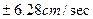b)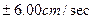c)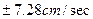d)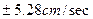Q-2 Find the displacement at t=2 sec

a) 7.0 cm

b) 6.5 cm

c) 6 cm

d) None of these

Q-3 Find the maximum acceleration and maximum velocity

a) 3.00 cm2/sec, 6.8 cm/sec

b) 3.56 cm2/sec, 6.0 cm/sec

c) 3.2 cm2/sec, 6.1 cm/sec

d) 3.56 cm2/sec, 6.8 cm/sec

Q-4 Find the equation of motion of the body

a)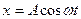b)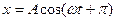c)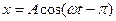d) None of these

Q-5  : Find the force acting on the body when t=2 sec

a) -64 dyne

b) -60 dyne

c) 0 dyne

d) None of these

Multiple Choice Questions

Q-6  A solid cylinder is attached to a horizontal massless spring so that it can roll with slipping along the horizontal surface. The spring constant is K .Mass of the cylinder is M.

The system is released from rest where the spring is stretched by x..The Center of mass of the cylinder execute SHM with time period T.Pick the correct value of T

a)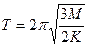b)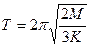c)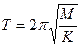d) None of these

Q-7   A mass M at the end of a spring executes SHM with a period t1 while the same mass execute SHM with a period t2 for another spring. T is the period of oscillation when the two springs are connected in series and Mass M is attached at the end.

Find out the correct relation

a)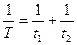b)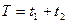c)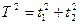d)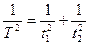Q-8 :

Consider a mass –spring  system.This system is given an  initial displacement  ,it begin to oscillate with frequency f1 .System is now bring to rest and again it is given different displacement  and f2 be its frequency of oscillation  then frequencies

a) f1 = f2

b) f1 > f2

c) f1 < f2

d) none of the above

Q-9: The instantaneous displacement of a particle of mass m executing SHM under a force constant k is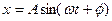Where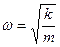The time average of kinetic energy over a Time period T is

a)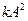b)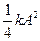c)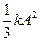d)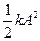Q-10:  For small amplitude of oscillations potential energy curve w.r.t distance travelled from equilibrium position is

a) Parabolic

b) Hyperbolic

c) Elliptical

d) circular

Q-11:

The homogenous linear differential equation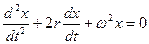Represents the equation of

a) Simple harmonic oscillator

b) Damped harmonic oscillator

c) Forced harmonic oscillator

d) None of the above

Q-12: Given the maximum velocity and acceleration of a harmonic oscillator as vmax and amax respectively, its time period in terms of vmax and amax is

a)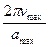b)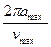c)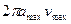d)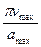Solutions

Note to our visitors :-

Thanks for visiting our website.